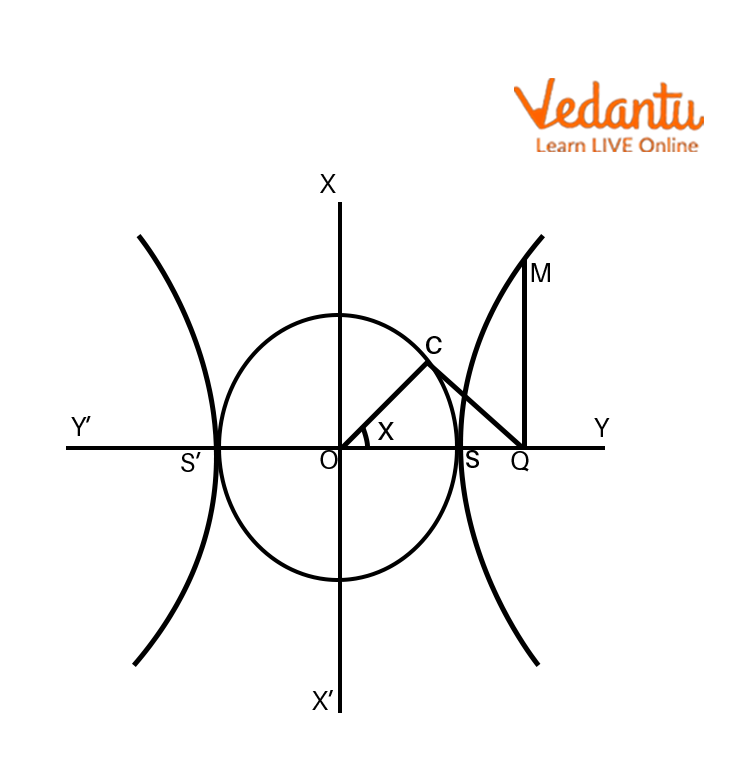Courses
Courses for Kids
Free study material
Free LIVE classes
More

# Parametric Form of HyperbolaLIVE
Join Vedantu’s FREE Mastercalss

## Parametric Equation of Hyperbola: Preface

Hyperbola is a subdivision of conic sections in the field of Mathematics. When the surface of a cone intersects a plane, curves are formed, and these curves are known as conic sections. There are three categories of conic sections: the eclipse, the hyperbola, and the parabola.

We use conic sections to study 3D geometry which has a vast number of applications in various fields of engineering. Also, when a spacecraft uses the gravitational slingshot technique, the path followed by the craft is a hyperbola. In this article, we will get to know about the parametric form of the hyperbola. We will also see some interesting facts about the hyperbola and also answer some of the questions.

## Parametric Coordinates of Hyperbola

In this topic, we will find how to calculate the parametric equation of the hyperbola.

To understand this in a simple way, let’s take the help of the following diagram.Parametric Coordinates of Hyperbola

The circle inscribed in between the hyperbola and on the transverse axis is called an auxiliary circle. If the equation of the hyperbola shown in the figure is $\dfrac{x^{2}}{a^{2}}-\dfrac{y^{2}}{b^{2}}=1$ then the equation of the auxiliary circle inscribed between the auxiliary circle will be $x^{2}+y^{2}=a^{2}$.

Let M$(x,y)$ be any point on the hyperbola $\dfrac{x^{2}}{a^{2}}-\dfrac{y^{2}}{b^{2}}=1$.

Now, from M make a line MQ to the transverse axis such that it is perpendicular to it and then select a point on the circle $x^{2}+y^{2}=a^{2}$ such that the angle OCQ is 90 degrees.

Now, join (C and Q) and (O and C), The length of OC is a. Now let the length ∠SOC,

Here the ∠SOC is called the eccentric angle of the point M on the hyperbola.

Now from the right triangle OCQ, we get

$\dfrac{OC}{OQ}=cos\Theta$

$\dfrac{a}{OQ}=\dfrac{1}{sec\Theta }$ , (The radius of the circle is a=OC)

$OQ=asec\Theta$

Therefore, the abscissa of the M(x,y) on the hyperbola is asecθ.

As the point M lies on the hyperbola $\dfrac{x^{2}}{a^{2}}-\dfrac{y^{2}}{b^{2}}=1$, so it will satisfy the equation .

So, $\dfrac{(asec\Theta )^{2}}{a^{2}}-\dfrac{y^{2}}{b^{2}}=1$ (as x = sec)

$\Rightarrow \dfrac{y^{2}}{b^{2}}=sec^{2}\Theta -1$

$\Rightarrow \dfrac{y^{2}}{b^{2}}=tan^{2}\Theta$

$\Rightarrow y^{2}=b^{2}tan^{2}\Theta$

$\Rightarrow y=btan\theta$

Hence, the coordinate of the point M is (asecθ,btanθ) and for all the values of θ This point lies on the hyperbola and hence the polar coordinates of a hyperbola is represented by $(asec\Theta ,btan\Theta )$.

## Parametric Form of Hyperbola

If we want to write a parametric form of the hyperbola, we can write it as

Case 1: if the hyperbola is horizontal then:

$f(t)=(x(t),y(t))$

$x(t)=asec\Theta$

$y(t)=btan\Theta$

Case 2: if the hyperbola is vertical then:

$f(t)=(x(t),y(t))$

$x(t)=atan\Theta$

$y(t)=asec\Theta$

## Interesting Facts

• When an object, let's say a jet, moves faster than the speed of sound it creates a conical form of a wave in space. When that wave intersects the ground, the curve we get from that intersection is a hyperbola.

• The cooling towers are generally made of hyperbolic shape to achieve 2 things; first, the least amount of material used to make it and second, the structure should be strong enough to withstand strong winds.

## Solved Examples

Q1. Find the parametric coordinates of the point $(3\sqrt{2},2)$ on the hyperbola $\dfrac{x^{2}}{9}-\dfrac{y^{2}}{4}=1$.

Ans The equation of the hyperbola is $\dfrac{x^{2}}{9}-\dfrac{y^{2}}{4}=1$.

If we compare it with general equation of hyperbola

$\dfrac{x^{2}}{a^{2}}-\dfrac{y^{2}}{b^{2}}=1$ we get

$a^{2}=9\Rightarrow a=3$

$b^{2}=4\Rightarrow b=2$

So the parametric coordinates of the hyperbola will be $(3sec\Theta ,2tan\Theta )$.

Now, to calculate the parametric coordinates,

$3sec\Theta =3\sqrt{2}\Rightarrow sec\Theta =\sqrt{2}\Rightarrow \Theta =45^{\circ}$

$2tan\Theta =2\Rightarrow tan\Theta =1\Rightarrow \Theta =45^{\circ}$

So the parametric coordinates will be $(3sec45^{\circ},2tan45^{\circ})$.

## Practice Questions

Question Write parametric for each of the hyperbolas below:

1. $\dfrac{(x-3)^{2}}{256}-\dfrac{(y+3)^{2}}{625}=1$

Ans:

$x=3+16sect$

$y=-5+25tant$

2. $\dfrac{(y+7)^{2}}{324}-\dfrac{(x-8)^{2}}{169}=1$

Ans:

$x=8+13sect$

$y=-7+18tant$

## Summary

The article summarises the concept of parametric coordinates of hyperbola and its form.

Now we know that if the equation a hyperbola is

$\dfrac{x^{2}}{a^{2}}-\dfrac{y^{2}}{b^{2}}=1$ then the parametric coordinates will be

$(x(t)=asec\Theta,y(t)=btan\Theta)$ where $\theta$ is the parameter.

Last updated date: 25th Sep 2023
Total views: 94.5k
Views today: 2.94k

## FAQs on Parametric Form of Hyperbola

1. What is the formula of the equation of parametric coordinates of a rectangular hyperbola?

If the equation of the hyperbola is  $xy=c^2$then the parametric coordinates of the hyperbola will be  $x(t)=c(t)$, $y(t)=\dfrac{c}{t}$.

2. What is the second parameter other than (asec,btan) that can be used in hyperbola?

The second parameter that can be used against $(x(t)=asec\Theta,y(t)=btan\Theta)$  is $(x(t)=acosec\Theta,y(t)=bcot\Theta)$ , they can be used if the equation of hyperbola is $\dfrac{x^{2}}{a^{2}}-\dfrac{y^{2}}{b^{2}}=1$ .

3. What is a rectangular hyperbola?

When the length of the major axis and minor axis of a hyperbola are equal, the hyperbola is known as a rectangular hyperbola.